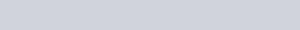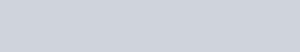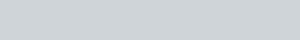# Loss functions in TensorFlow 2.0

In this tutorial, we will learn about several types of loss functions and their implementation in Python using TensorFlow.

## What is the loss function?

The loss function is often referred to as the error function or the cost function. These are errors caused by machines while training data, and by utilizing an optimizer and modifying weight machines, you may limit the loss and accurately predict results.

The formula for calculating loss :

Loss= abs(Y_pred – Y_actual)

Inputs required by the loss function are as follows :

• y_true (true label): Can either be 0 or 1.
• y_pred (predicted value): It is the predicted label from the model i.e, a single floating-point value which represents either a logit, (i.e, value that ranges from [-inf to inf] when from_logits=True) or a probability (i.e, value range in between [0., 1.] when from_logits=False).

Keras and TensorFlow both provide loss function documentation, but with the same code, which you can get here:

## Types of Loss Function

• ### Binary Cross-Entropy loss

The cross-entropy between true labels and anticipated outputs is calculated using binary cross-entropy. It is used when the two-class issues arise like (yes or no, failure or success,0 or 1, head or tail when tossing a random coin).

First of all Importing TensorFlow library to calculate the different types of loss.

Check the TensorFlow version.

```import tensorflow as tf
print(tf.__version__)```

Output

`2.7.0`

Let’s see how to implement binary cross-entropy in Python.

```# Input values
y_true=[[0.,1.],[1.,0.]]
# Predicted
y_pred=[[0.4,0.5],[0.3,0.6]]

#Binary-Cross-entropy
binary_cross=tf.keras.losses.BinaryCrossentropy()
binary_cross(y_true=y_true,y_pred=y_pred).numpy()```

In the above code, we take batch size = 2 i.e y_true, and number of samples = 4 i.e y_pred

Output

`0.83105886`

This is how we can compute binary cross-entropy.

• ### Categorical Crossentropy

To compute the loss between true and predicted labels, the categorical cross-entropy loss function is utilized. It’s mostly used to solve problems with multiclass categorization. For example Image Classification of human body parts like ear, nose, knee, belly, etc.

Let’s see how to implement Categorical Crossentropy.

```# Actual
y_true = [[0, 0, 1], [0, 1, 0]]
#Predicted
y_pred = [[0.04, 0.90, 0.50], [0.1, 0.4, 0.1]]
# Cat cross loss
cat_cross_entropy = tf.keras.losses.CategoricalCrossentropy()
cat_cross_entropy(y_true=y_true,y_pred=y_pred).numpy()```

In the above code, we take batch size=2 i.e  y_true and num_of_samples=8 i.e y_pred

Output

`1.1308815`

That’s how we can calculate categorical cross-entropy.

• ### Sparse Categorical Crossentropy loss

When there are two or more classes in our classification work, Sparse Categorical Crossentropy is employed. However, there is one distinction between categorical and sparse categorical cross-entropy: sparse categorical cross-entropy labels are supposed to be supplied in integers.

Let’s see the implementation of sparse categorical cross-entropy.

```#iActual label
y_true = [1, 2]
#Predicted Labl
y_pred = [[0.07, 0.90, 0],
[0.1, 0.6, 0.1]]
#Sparse Categorical-Crossentropy
tf.keras.losses.sparse_categorical_crossentropy(y_true,y_pred).numpy()```

Output

`array([0.07490139, 2.0794415 ], dtype=float32)`

That’s how we can calculate sparse categorical cross-entropy.

• ### Poisson Loss

It is the average of Tensors elements. Poisson loss can be calculated using the formula:

y_pred – y_true*log(y_true)

Let’s see how to implement Poisson loss in python :

```y_true = [[1., 0.], [0., 0.]]
#Predicted
y_pred = [[1., 0.], [0., 0.]]
# Poisson loss
pois = tf.keras.losses.Poisson()
pois(y_true, y_pred).numpy()
```

Output

`0.24999997`
• ### Kullback-Leibler Divergence Loss

It’s also known as KL divergence, and it’s determined by taking the negative sum of each event’s probability P and multiplying it by the log of the likelihood of that event.

FormulaLet’s see how to implement KL Divergence loss using TensorFlow.

```y_true = [[0, 1], [0, 0]]
y_pred = [[0.4, 0.6], [0.6, 0.4]]
# The reduction type 'auto'/'sum over batch size' is being used.
kl = tf.keras.losses.KLDivergence()
kl(y_true, y_pred).numpy()
```

Output

`0.2554105`
• ### Mean Squared Error (MSE)

The MSE value indicates how near a regression line is to the anticipated points. And it’s as simple as squaring the distance between the spot and the regression line. We employ square to solve the problem of the negative sign.

FormulaMSE value lies in between 0 to infinity. A smaller value of MSE indicates the best performance of the model.

MSE is sensitive to outliers.

Implementation for MSE:

```#Actual
y_true = [[9., 9.],
[0., 0.]]
#Predicted
y_pred = [[9., 9.],
[1., 0.]]
#MSE
mse = tf.keras.losses.MeanSquaredError()
mse(y_true, y_pred).numpy()```

Output

`0.25`

• ### Mean Absolute Error(MAE)

The MAE is calculated by calculating the distance between a point and the regression line. MAE is also sensitive to outliers just like MSE, always remove all outliers in data when you use MAE.

FormulaImplementation for MAE:

```# Input values
y_true = [[10., 15.],
[20., 25.]]
# Predicted values
y_pred = [[10., 15.],
[20., 0.]]
#MAE calculation
mae = tf.keras.losses.MeanAbsoluteError()
mae(y_true, y_pred).numpy()```

Output

`6.25`
• ### Huber Loss

The Huber loss is the mixture of ( MSE+MAE). Huber loss is robust to outliers.

For small values, the Huber loss function is quadratic, whereas, for bigger values, it is linear.

FormulaLet’s see the implementation of Huber loss in python:

```# Actual values
y_true = [[15., 20.],
[25., 30.]]
# Predicted value
y_pred = [[15., 20.],
[25., 0.]]
#Huber loss
h_loss = tf.keras.losses.Huber()
h_loss(y_true, y_pred).numpy()```

Output

`7.375`
• ### Hinge Loss

Hinge loss is used by Support Vector Machines(SVM) to solve problems like “maximum margin” classification.

The value of y_true in Hinge loss is [-1 or 1].In Hinge loss binary value i.e 0 or 1 converted into -1 or 1.

FormulaImplementation of Hinge loss using TensorFlow:

```#Actual value
y_true = [[1., 0.], [1., 0.]]
#Predicted value
y_pred = [[0.4, 0.5], [0.5, 0.4]]
#Hinge loss
h_loss = tf.keras.losses.Hinge()
h_loss(y_true, y_pred).numpy()```

Output

`1.0`